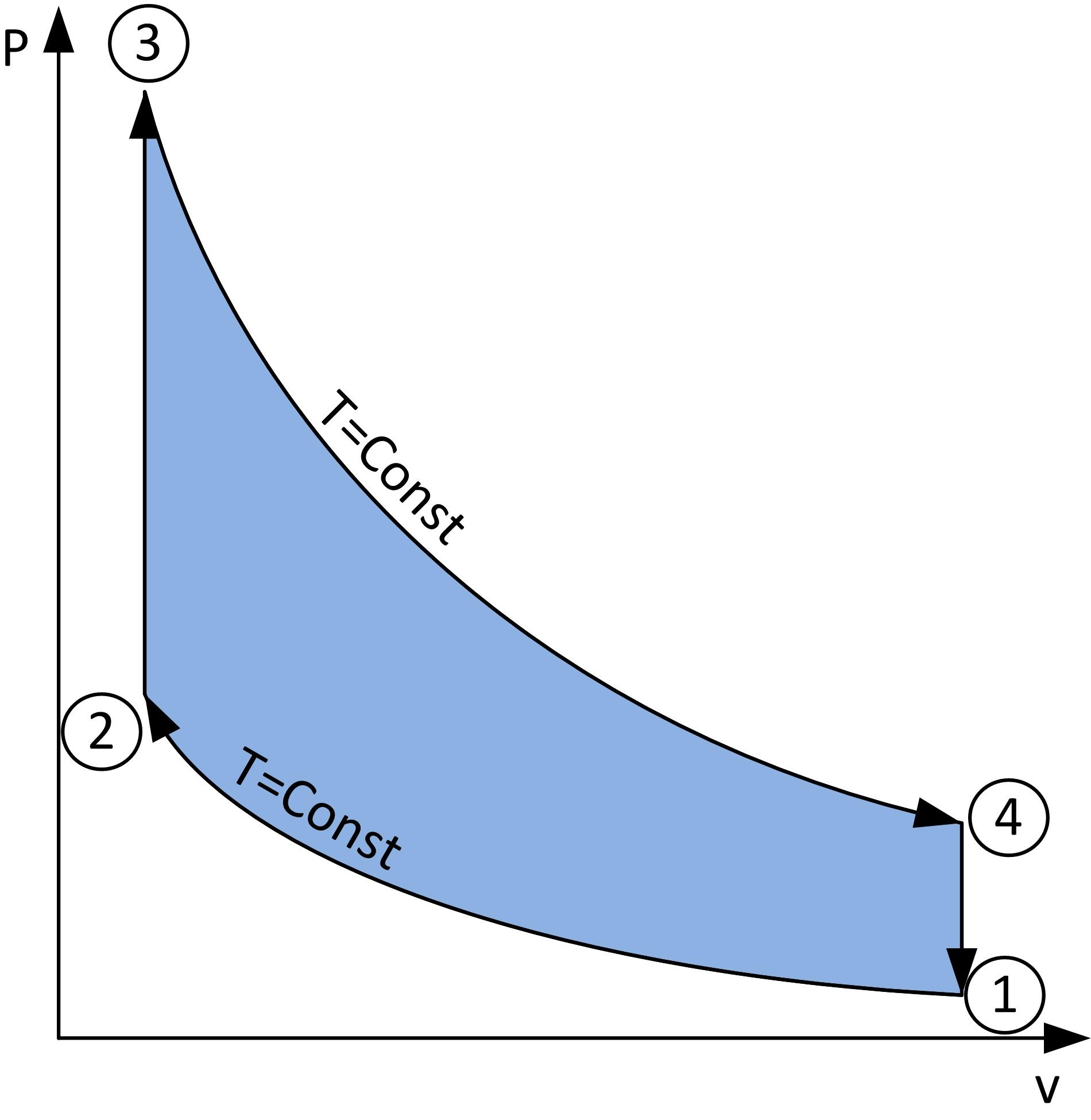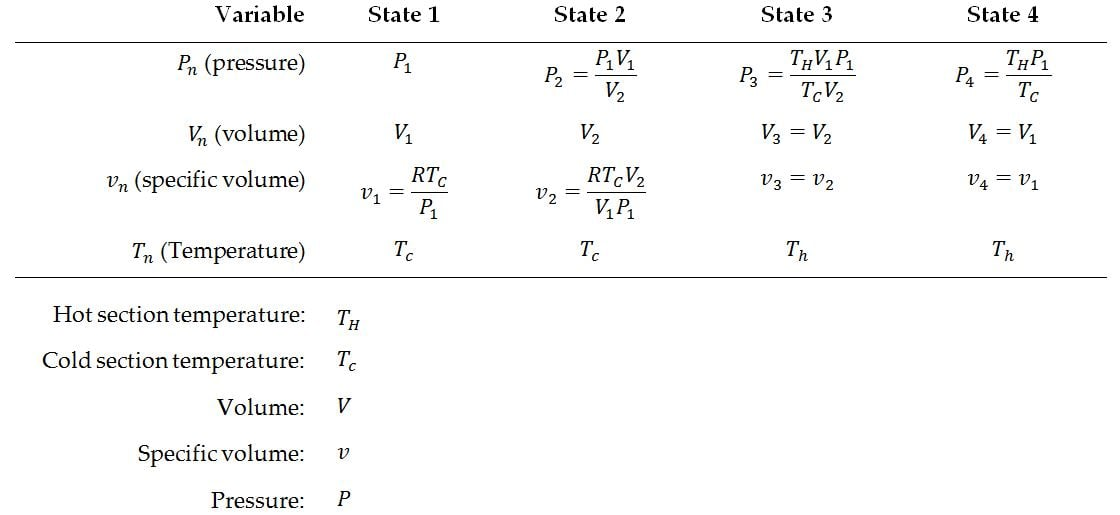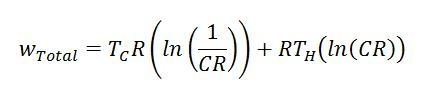# Ideal Stirling Cycle Calculator

## Calculator Inputs

 Hot end temperature (K): Cold end temperature (K): Compression Ratio: Specific gas constant of working fluid (J/kg-K): Pressure at state 1 (kPa): Volume at state 1 (cc): Amount of heat applied to engine (W):

## Calculator Outputs

 Predicted Frequency (RPM) Predicted Power Out (W) Predicted Efficiency (0-1) Predicted Average Pressure (kPa) Swept Volume (cc) Mass of Working Fluid (mg) Work Out Per Cycle (J) Predicted Specific Work Out Per Cycle (J/mg-working fluid) Volume at State 1 (cc) Absolute Pressure at State 1 (kPa) Temperature at State 1 (K) Volume at State 2 (cc) Absolute Pressure at State 2 (kPa) Temperature at State 2 (K) Volume at State 3 (cc) Absolute Pressure at State 3 (kPa) Temperature at State 3 (K) Volume at State 4 (cc) Absolute Pressure at State 4 (kPa) Temperature at State 4 (K)

# Summary of EquationsFigure: A loop showing the relation between pressure and volume during a cycle of the Stirling engine, and with each state labelled

It’s possible to calculate the fluid properties at all these different states with the following formulas:Additionally, useful work produced can be calculated with the following formula. CR stands for “Compression Ratio” (max engine volume divided by min engine volume). Please note that this formula gives the amount of work per unit mass of working fluid per revolution of the Stirling engine. Temperatures must also be in an absolute scale (i.e. Rankine or Kalvin).Equation 5: Work per unit mass of working fluid delivered by an Ideal Stirling engine per revolution (cycle)

It’s important to keep in mind that all these numbers presented are for the ideal Stirling cycle, which will never exist in real life, all real engines are approximations of the ideal thermodynamic cycles. Knowing how to modify these ideal relations to reflect the real world is a whole other topic, one that might be treated in a future article!